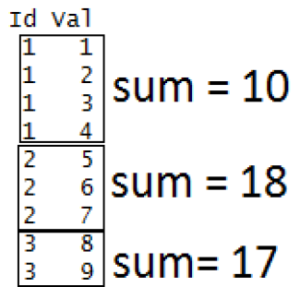# Looping over Objects in R Programming

• Last Updated : 02 Aug, 2020

Prerequisite: Data Structures in R Programming

One of the biggest issues with the “for” loop is its memory consumption and its slowness in executing a repetitive task. And when it comes to dealing with large data set and iterating over it, for loop is not advised. R provides many alternatives to be applied to vectors for looping operations that are pretty useful when working interactively on a command line. In this article, we deal with `apply()` function and its variants:

`apply()lapply()sapply()tapply()mapply()`

Let us see what each of these functions does.

Looping FunctionOperation
`apply()`Applies a function over the margins of an array or matrix
`lapply()`Apply a function over a list or a vector
`sapply()`Same as `lapply()` but with simplified results
`tapply()`Apply a function over a ragged array
`mapply()`Multivariate version of `lapply()`
• `apply()`: This function applies a given function over the margins of a given array.

apply(array, margins, function, …)

array = list of elements
margins = dimension of the array along which the function needs to be applied
function = the operation which you want to perform

Example:

 `# R program to illustrate``# apply() function`` ` `# Creating a matrix``A ``=` `matrix(``1``:``9``, ``3``, ``3``)``print``(A)`` ` `# Applying apply() over row of matrix``# Here margin 1 is for row ``r ``=` `apply``(A, ``1``, ``sum``)``print``(r)`` ` `# Applying apply() over column of matrix``# Here margin 2 is for column``c ``=` `apply``(A, ``2``, ``sum``)``print``(c)`

Output:

```[, 1] [, 2] [, 3]
[1, ]    1    4    7
[2, ]    2    5    8
[3, ]    3    6    9

 12 15 18
  6 15 24
```
• `lapply():` This function is used to apply a function over a list. It always returns a list of the same length as the input list.

lapply(list, function, …)

list = Created list
function = the operation which you want to perform

Example:

 `# R program to illustrate``# lapply() function`` ` `# Creating a matrix``A ``=` `matrix(``1``:``9``, ``3``, ``3``)`` ` `# Creating another matrix``B ``=` `matrix(``10``:``18``, ``3``, ``3``) `` ` `# Creating a list``myList ``=` `list``(A, B)`` ` `# applying lapply()``determinant ``=` `lapply(myList, det)``print``(determinant)`

Output:

```[]
 0

[]
 5.329071e-15
```
• `sapply():` This function is used to simplify the result of `lapply()`, if possible. Unlike `lapply()`, the result is not always a list. The output varies in the following ways:-
• If output is a list containing elements having length 1, then a vector is returned.
• If output is a list where all the elements are vectors of same length(>1), then a matrix is returned.
• If output contains elements which cannot be simplified or elements of different types, a list is returned.

sapply(list, function, …)

list = Created list
function = the operation which you want to perform

Example:

 `# R program to illustrate``# sapply() function`` ` `# Creating a list``A ``=` `list``(a ``=` `1``:``5``, b ``=` `6``:``10``)`` ` `# applying sapply()``means ``=` `sapply(A, mean)``print``(means)`

Output:

```a b
3 8
```

A vector is returned since the output had a list with elements of length 1.

• `tapply()`: This function is used to apply a function over subset of vectors given by a combination of factors.

tapply(vector, factor, function, …)

vector = Created vector
factor = Created factor
function = the operation which you want to perform

Example:

 `# R program to illustrate``# tapply() function`` ` `# Creating a factor``Id` `=` `c(``1``, ``1``, ``1``, ``1``, ``2``, ``2``, ``2``, ``3``, ``3``)`` ` `# Creating a vector``val ``=` `c(``1``, ``2``, ``3``, ``4``, ``5``, ``6``, ``7``, ``8``, ``9``)`` ` `# applying tapply()``result ``=` `tapply(val, ``Id``, ``sum``)``print``(result)`

Output:

```1  2  3
10 18 17
```

How does the above code work?• `mapply()`: It’s a multivariate version of `lapply()`. This function can be applied over several list simultaneously.

mapply(function, list1, list2, …)

function = the operation which you want to perform

list1, list2 = Created lists

Example:

 `# R program to illustrate``# mapply() function`` ` `# Creating a list``A ``=` `list``(c(``1``, ``2``, ``3``, ``4``))`` ` `# Creating another list``B ``=` `list``(c(``2``, ``5``, ``1``, ``6``))`` ` `# Applying mapply()``result ``=` `mapply(``sum``, A, B)``print``(result)`

Output:

``` 24
```

My Personal Notes arrow_drop_up